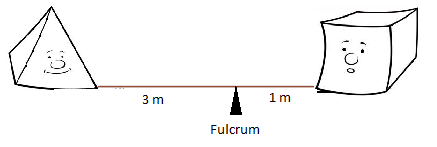# [MPG] Mass Point Geometry : Tricks for Triangles for CAT Exam

[MPG] Mass Point Geometry : Tricks for Triangles for CAT Exam
4.5 (90%) 8 vote[s]

## Introduction to Mass Point Geometry [MPG]

Mass point Geometry (MPG) is a technique borrowed from the concepts of physics which we apply to solve many complex problems involving triangles and intersecting lines. The technique is easy to understand and apply.

MPG technique also simplifies calculations of ratios in geometric figures in an intuitive way by merging algebra, geometry, and basic physics. The method is as simple as balancing a see-saw.The basic idea is that of a see-saw with weights at each end. The see-saw will balance if the product of the mass and its distance to the fulcrum is the same for each mass.For example, if a square pyramid is 3 m from the fulcrum, then a cube of the same base must be located 1 m on the other side of the fulcrum for the see-saw to balance.

NOTE: Square pyramid of the same base as that of a cube has 1/3rd of the volume of square hence, the weight is also in the ratio 1:3 say x: 3x. Total weight at the pivot = 3x+x = 4x.

Distance × Weight = (3m)(x) = (1m)(3x)  i.e., distance weight product = 3mx on both sides of the fulcrum therefore the see-saw is balanced.

To make you understand it better, watch the below video.

### Mass Point Geometry Solved Examples

Example 01:

Example 02: